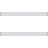# Alembic in 2021 (part3)

• Alembic
• bash
• Data Science
• en
• english
• ETL
• geospatial
• GIS
• h3
• hexagons
• Jupyter
• PostGIS
• uber
• Par Tarek Baaga, le 23 juin 2021

In the last tutorials, we experimented with the basics of Alembic. In this tutorial, we are going to take it up a notch by creating and migrating functions with Alembic Utils.

### Alembic Utils

This extension is used with Alembic to make the creation of function, views, or trigger a very simple and fast process.

### Create a Function

``````from alembic import op
from alembic_utils.pg_function import PGFunction``````

``````def upgrade():
public_to_upper = PGFunction(
schema="public",
signature="createbuffer(integer)",
definition="""
RETURNS TABLE(buffercreated geometry)
LANGUAGE sql
AS \$function\$
select ST_Transform(ST_Buffer(ST_Transform(geom, 3857), \$1), 4326)
from public.geomtable
\$function\$
;
"""
)

op.create_entity(public_to_upper)

public_to_upper = PGFunction(
schema="public",
signature="createbuffer(integer)",
definition="# Not Used"
)

op.drop_entity(public_to_upper)``````

The function created lets you add a buffer around the point of the geomtable created previously.

Another interesting thing to try is to migrate the created function from the public schema to the tiger schema for example:

Before inserting anything in the upgrade and downgrade functions, we have to define the targeted function and store it in a list that may contain one or multiple functions:

``````bufferfunction = {
'signature': """createbuffer(integer)""",
'definition': """
RETURNS TABLE(buffercreated geometry)
LANGUAGE sql
AS \$function\$
select ST_Transform(ST_Buffer(ST_Transform(geom, 3857), \$1), 4326)
from public.geomtable
\$function\$
;
"""
}

list_of_function_descriptions_to_migrate = [
bufferfunction
]

list_of_functions_in_public = [
PGFunction(
schema='public',
signature=f['signature'],
definition=f['definition']) for f in
list_of_function_descriptions_to_migrate]
list_of_functions_in_tiger = [
PGFunction(schema='tiger',
signature=f['signature'],
definition=f['definition']) for f in
list_of_function_descriptions_to_migrate]``````

All we have to do now is iterate over the buffer function dictionary to move the function to the tiger schema or send it back in public. This method can scale up very well when there are multiple functions to migrate all at once:

``````def upgrade():
for function_in_public in list_of_functions_in_public:
op.drop_entity(function_in_public)
for function_in_tiger in list_of_functions_in_tiger:
op.create_entity(function_in_tiger)

for function_in_tiger in list_of_functions_in_tiger:
op.drop_entity(function_in_tiger)
for function_in_public in list_of_functions_in_public:
op.create_entity(function_in_public)``````

### The basics of using hexagons

The first thing to do on top of the PostGIS extension is to add the pgh3 extension in a new alembic revision. In the second article, we added the ph3 extension to be able to add the h3. (For more information about hexagons, check out our blog post):

https://anagraph.io/en/articles/hexagons-start-with-why/

`````` op.execute(
'create extension IF NOT EXISTS pgh3'
)``````

We developed a schema inside our team that we go through every time we are about to use hexagons:

Now that we added the extension pgh3, we can use the shapefile of the table Quartier sociologiques 2014 to generate hexagons from the geom like this:

``````with envelope as (
select st_envelope(geom) geom from "Quartiers_sociologiques_2014"
), h3_id as (
select h3_polyfill(geom, 8) id from envelope
), h3 as (
select id, st_setsrid(h3_h3index_to_geoboundary(id), 4326) geom from h3_id
)
select h3.id, h3.geom from h3``````

The query is divided into three steps. The first one is to create a bounding box that surrounds each geometry. The second step is to use the h3_polyfill function and choose a resolution (8 in this case). This second step gives us the H3 index but without the geometry. If we want the geometry of the hexagons we have to use h3_h3index_to_geoboundary function that convert the h3 index into polygon coordinates. In this case, we add a special projection with the PostGIS function st_setsrid. You can read more about h3 function here:

https://github.com/dlr-eoc/pgh3/blob/master/doc/pgh3.md

### Create hexagons with function

Now let’s use the query for the hexagons to create a function where we can choose the resolution of the hexagons. The next step is to add the function in the Alembic file:

``````def upgrade():
create_hexes = PGFunction(
schema="public",
signature="create_hexes(resolution integer)",
definition="""
RETURNS TABLE(hexa_id text, hexa_geom geometry)
LANGUAGE plpgsql
AS \$function\$
begin
return query
with envelope as (
select st_envelope(geom) geom from "Quartiers_sociologiques_2014"
), h3_id as (
select h3_polyfill(geom, resolution) id from envelope
), h3 as (
select id, st_setsrid(h3_h3index_to_geoboundary(id), 4326) geom from h3_id
)
select h3.id, h3.geom from h3;
end;\$function\$
;
"""
)

op.create_entity(create_hexes)

create_hexes = PGFunction(
schema="public",
signature="create_hexes(resolution integer)",
definition="# Not Used"
)

op.drop_entity(create_hexes)``````

By using this function and choosing the resolution 8 as a parameter we can see the hexagons like this:

### Aggregate points onto hexes

Until now, we saw how to transform polygons into hexagons but what about the point data type?

By generating the hexagons first by using the table ”Quartiers_sociologiques_2014″, we are able to generate hexagons for the table representing the location of outdoor Wi-Fi coverage areas based in Montreal (mtlwifi_bornes)

The query is broken down as the following:

``````with envelope as (
select st_envelope(geom) geom from public."Quartiers_sociologiques_2014"
), h3_id as (
select h3_polyfill(geom, 8) id from envelope
), h3 as (
select id, st_setsrid(h3_h3index_to_geoboundary(id), 4326) geom
from h3_id
), p as (
select geom, "type" from mtlwifi_bornes as p
)
select h3.id, h3.geom, p."type" from h3, p
where h3.geom && p.geom and st_dwithin(ST_transform(h3.geom, 3857), ST_transform(p.geom, 3857), 150)
group by h3.id, h3.geom, p."type"``````

First of all the query is creating hexagons, and then by using the spatial function st_dwithin we are getting the number of wifi networks that are within 150m. (Don’t forget to project your data into EPSG:3857 to be able to use meters). Instead of using ST_Intersects with a buffer, we used ST_DWithin to simplify the query. The result obtained will look like this:

If you are interested in seeing another use case of hexagons applied this time to real estate, check out our blog post here: https://anagraph.io/en/articles/hexagons-start-with-why/

### Alembic automation

Until now, we saw how to upgrade the alembic files manually, but we could add another service in the docker-compose file to add the python dependency and to run the alembic upgrade command:

``````osgeo:
build:
context: .
dockerfile: osgeo.Dockerfile
environment:
EPSG_CODE: 4326
depends_on:
- db
volumes:
- ./geodata:/geodata
- ./etl:/etl